# Decay Schedules¶

Classes for defining decay schedules for simulated annealing.

class GeomDecay(init_temp=1.0, decay=0.99, min_temp=0.001)[source]

Schedule for geometrically decaying the simulated annealing temperature parameter T according to the formula: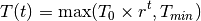where:

•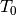is the initial temperature (at time t = 0);
•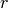is the rate of geometric decay; and
•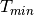is the minimum temperature value.
Parameters: init_temp (float, default: 1.0) – Initial value of temperature parameter T. Must be greater than 0. decay (float, default: 0.99) – Temperature decay parameter, r. Must be between 0 and 1. min_temp (float, default: 0.001) – Minimum value of temperature parameter. Must be greater than 0.

Example

>>> import mlrose
>>> schedule = mlrose.GeomDecay(init_temp=10, decay=0.95, min_temp=1)
>>> schedule.evaluate(5)
7.73780...

evaluate(t)[source]

Evaluate the temperature parameter at time t.

Parameters: t (int) – Time at which the temperature paramter T is evaluated. temp (float) – Temperature parameter at time t.
class ArithDecay(init_temp=1.0, decay=0.0001, min_temp=0.001)[source]

Schedule for arithmetically decaying the simulated annealing temperature parameter T according to the formula: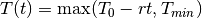where:

•is the initial temperature (at time t = 0);
•is the rate of arithmetic decay; and
•is the minimum temperature value.
Parameters: init_temp (float, default: 1.0) – Initial value of temperature parameter T. Must be greater than 0. decay (float, default: 0.0001) – Temperature decay parameter, r. Must be greater than 0. min_temp (float, default: 0.001) – Minimum value of temperature parameter. Must be greater than 0.

Example

>>> import mlrose
>>> schedule = mlrose.ArithDecay(init_temp=10, decay=0.95, min_temp=1)
>>> schedule.evaluate(5)
5.25

evaluate(t)[source]

Evaluate the temperature parameter at time t.

Parameters: t (int) – Time at which the temperature paramter T is evaluated. temp (float) – Temperature parameter at time t.
class ExpDecay(init_temp=1.0, exp_const=0.005, min_temp=0.001)[source]

Schedule for exponentially decaying the simulated annealing temperature parameter T according to the formula: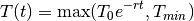where:

•is the initial temperature (at time t = 0);
•is the rate of exponential decay; and
•is the minimum temperature value.
Parameters: init_temp (float, default: 1.0) – Initial value of temperature parameter T. Must be greater than 0. exp_const (float, default: 0.005) – Exponential constant parameter, r. Must be greater than 0. min_temp (float, default: 0.001) – Minimum value of temperature parameter. Must be greater than 0.

Example

>>> import mlrose
>>> schedule = mlrose.ExpDecay(init_temp=10, exp_const=0.05, min_temp=1)
>>> schedule.evaluate(5)
7.78800...

evaluate(t)[source]

Evaluate the temperature parameter at time t.

Parameters: t (int) – Time at which the temperature paramter T is evaluated. temp (float) – Temperature parameter at time t.
class CustomSchedule(schedule, **kwargs)[source]

Class for generating your own temperature schedule.

Parameters: schedule (callable) – Function for calculating the temperature at time t with the signature schedule(t, **kwargs). kwargs (additional arguments) – Additional parameters to be passed to schedule.

Example

>>> import mlrose
>>> def custom(t, c): return t + c
>>> kwargs = {'c': 10}
>>> schedule = mlrose.CustomSchedule(custom, **kwargs)
>>> schedule.evaluate(5)
15

evaluate(t)[source]

Evaluate the temperature parameter at time t.

Parameters: t (int) – Time at which the temperature paramter T is evaluated. temp (float) – Temperature parameter at time t.